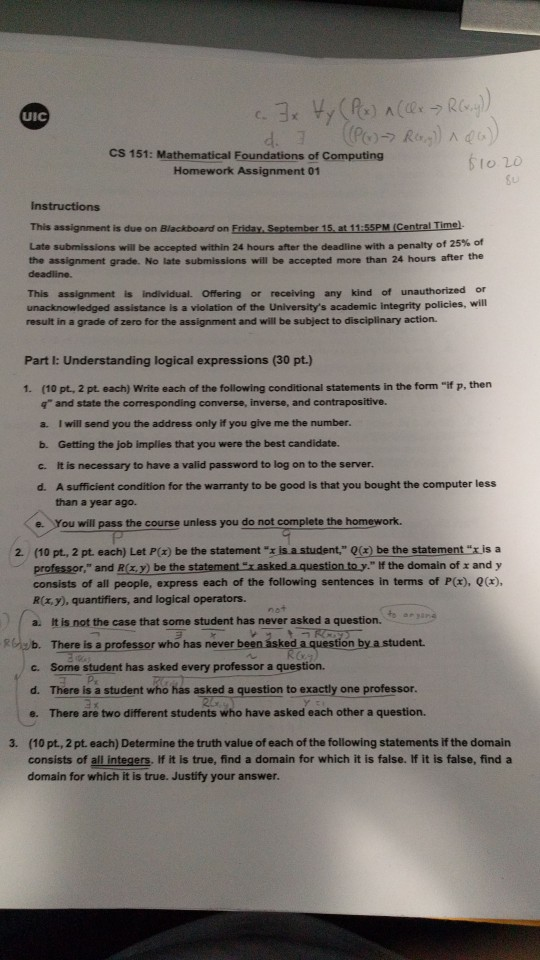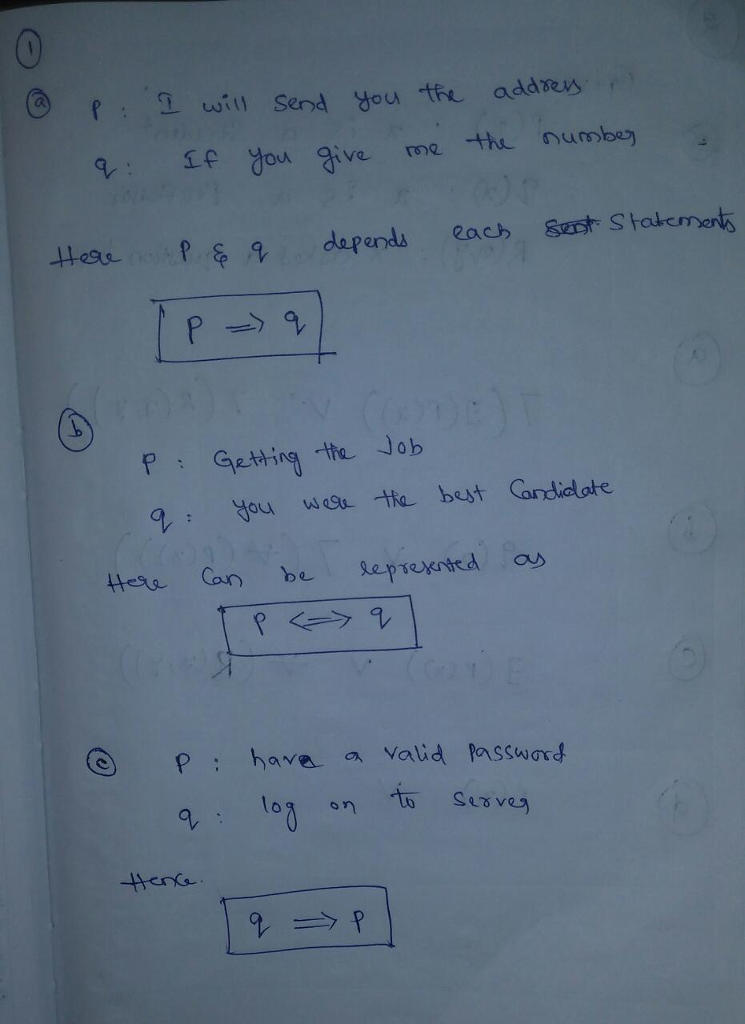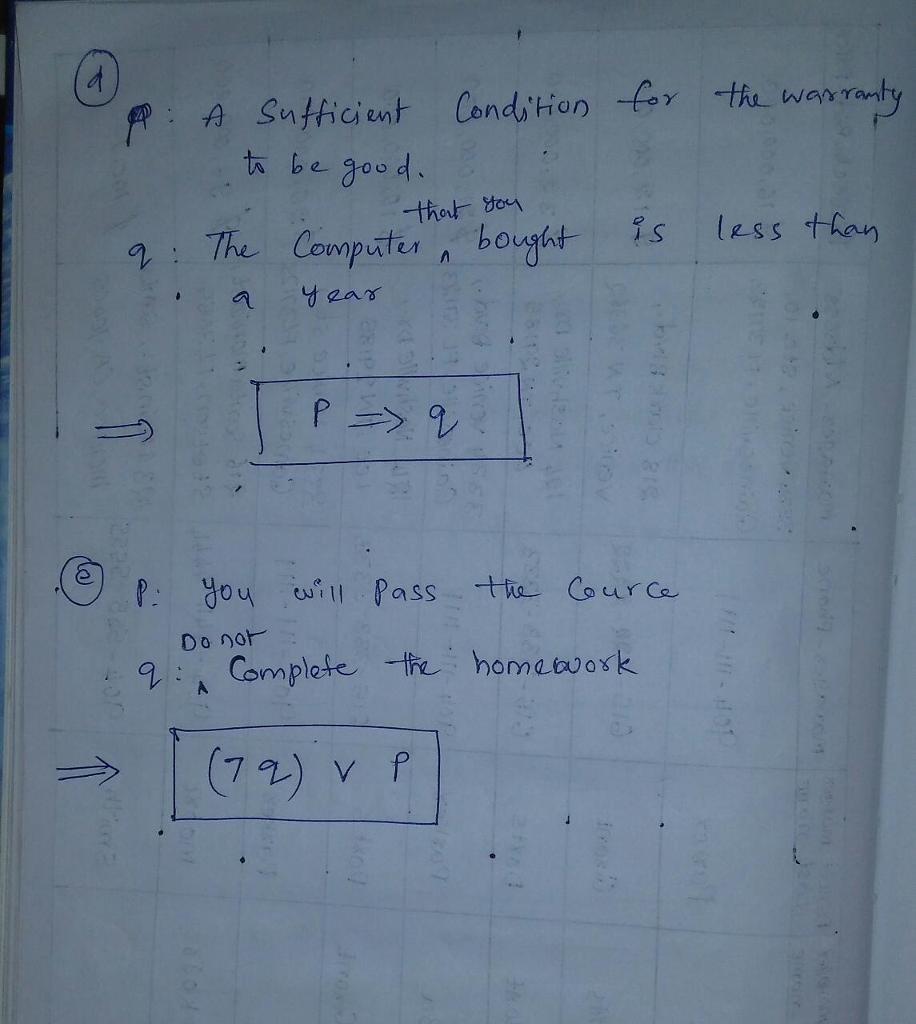# Question & Answer: Write each of the following conditional statements in the form "if p, then q" and state the corresponding converse, inver…..Write each of the following conditional statements in the form “if p, then q” and state the corresponding converse, inverse, and contrapositive. a. I will send you the address only if you give me the number. b. Getting the job implies that you were the best candidate. c. It is necessary to have a valid password to log on to the server. d. A sufficient condition for the warranty to be good is that you bought the computer less than a year ago. e. You will pass the course unless you do not complete the homework. Let P(x) be the statement “x is a student,” Q(x) be the statement “x is a professor,” and R(x, y) be the statement.” x asked a question to y.” If the domain of x and y consists of all people, express each of the following sentences in terms of P(x), Q(x), R(x, y), quantifiers, and logical operators. a. It is not the case that some student has never asked a question. b. There is a professor who has never been asked a question by a student. c. Some student has asked every professor a question. d. There is a student who has asked a question to exactly one professor. e. There are two different students who have asked each other a question. Determine the truth value of each of the following statements if the domain consists of all integers. If it is true, find a domain for which it is false. If it is false, find a domain for which it is true. Justify your answer.